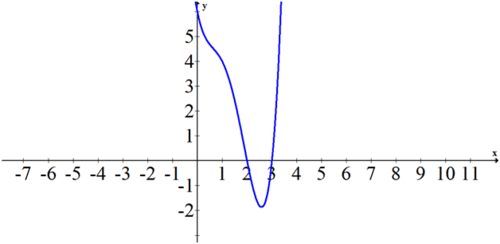# 11.1 Fundamental Theorem of Algebra

$$\newcommand{\vecs}{\overset { \rightharpoonup} {\mathbf{#1}} }$$ $$\newcommand{\vecd}{\overset{-\!-\!\rightharpoonup}{\vphantom{a}\smash {#1}}}$$$$\newcommand{\id}{\mathrm{id}}$$ $$\newcommand{\Span}{\mathrm{span}}$$ $$\newcommand{\kernel}{\mathrm{null}\,}$$ $$\newcommand{\range}{\mathrm{range}\,}$$ $$\newcommand{\RealPart}{\mathrm{Re}}$$ $$\newcommand{\ImaginaryPart}{\mathrm{Im}}$$ $$\newcommand{\Argument}{\mathrm{Arg}}$$ $$\newcommand{\norm}{\| #1 \|}$$ $$\newcommand{\inner}{\langle #1, #2 \rangle}$$ $$\newcommand{\Span}{\mathrm{span}}$$ $$\newcommand{\id}{\mathrm{id}}$$ $$\newcommand{\Span}{\mathrm{span}}$$ $$\newcommand{\kernel}{\mathrm{null}\,}$$ $$\newcommand{\range}{\mathrm{range}\,}$$ $$\newcommand{\RealPart}{\mathrm{Re}}$$ $$\newcommand{\ImaginaryPart}{\mathrm{Im}}$$ $$\newcommand{\Argument}{\mathrm{Arg}}$$ $$\newcommand{\norm}{\| #1 \|}$$ $$\newcommand{\inner}{\langle #1, #2 \rangle}$$ $$\newcommand{\Span}{\mathrm{span}}$$$$\newcommand{\AA}{\unicode[.8,0]{x212B}}$$

You have learned that a quadratic has at most two real zeroes and a cubic has at most three real zeros. You may have noticed that the number of real zeros is always less than or equal to the degree of the polynomial. By looking at a graph you can see when a parabola crosses the $$x$$ axis 0,1 or 2 times, but what does this have to do with complex numbers?

## The Fundamental Theorem of Algebra

A real number is any rational or irrational number. When a real number is squared, it will always produce a non-negative value. Complex numbers include real numbers and another type of number called imaginary numbers. Unlike real numbers, imaginary numbers may produce a negative value when squared. The square root of negative one is defined to be the imaginary number $$i$$.

$$i=\sqrt{-1}$$ and $$i^{2}=-1$$

Complex numbers are written with a real component and an imaginary component. All complex numbers can be written in the form $$a+b i$$. When the imaginary component is zero, the number is simply a real number. This means that real numbers are a subset of complex numbers.

The Fundamental Theorem of Algebra states that an $$n^{t h}$$ degree polynomial with real or complex coefficients has, with multiplicity, exactly $$n$$ complex roots. This means a cubic will have exactly 3 roots, some of which may be complex.

Take the following polynomial.

$$f(x)=x^{2}+9$$

At first you may think that this does not have any roots but the Fundamental Theorem of Algebra states that it must have 2 roots. Both roots for this polynomial are complex.

To find the roots for this complex polynomial, set $$y=0$$ and solve for $$x$$. This will give you the two zeros.

\begin{aligned} 0 &=x^{2}+9\\-9 &=x^{2} \\ \pm 3 i &=x \end{aligned}

Thus the linear factorization of the function is:

$$f(x)=(x-3 i)(x+3 i)$$

Multiplicity refers to when a root counts more than once. For example, in the following function the only root occurs at $$x=3$$.

$$f(x)=(x-3)^{2}$$

The Fundamental Theorem of Algebra states that this $$2^{\text {nd }}$$ degree polynomial must have exactly 2 roots with multiplicity. This means that the root $$x=3$$ has multiplicity 2 . One way to determine the multiplicity is to simply look at the degree of each of the factors in the factorized polynomial.

$$g(x)=(x-1)(x-3)^{4}(x+2)$$

This function has 6 roots. The first two roots $$x=1$$ and $$x=-2$$ have multiplicities of 1 because the power of each of their binomial factors is 1 . The third root $$x=3$$ has a multiplicity of 4 because the power of its binomial factor is 4 . Keep in mind that all polynomials can be written in factorized form like the above polynomial, due to a theorem called the Linear Factorization Theorem.

## Examples

##### Example 1

Earlier, you were asked about the graphs of parabolas and their relationships to the zeroes of the parabolas. When a parabola fails to cross the $$x$$ axis it still has 2 roots. These two roots happen to be imaginary numbers. The function $$f(x)=x^{2}+4$$ does not cross the $$x$$ axis, but its roots are $$x=\pm 2 i$$.

##### Example 2

Identify the polynomial that has the following five roots. $$x=0,2,3, \pm \sqrt{5} i$$

Write the function in factorized form.

$$f(x)=(x-0)(x-2)(x-3)(x-\sqrt{5} i)(x+\sqrt{5} i)$$

When you multiply through, it will be helpful to do the complex conjugates first. Remember that Complex conjugates are pairs of complex numbers with real parts that are identical and imaginary parts that are of equal magnitude but opposite signs. The complex conjugates in this equation are $$(x-\sqrt{5} i)(x+\sqrt{5} i)$$.

$$f(x)=x\left(x^{2}-5 x+6\right)\left(x^{2}-5 \cdot(-1)\right)$$

$$f(x)=\left(x^{3}-5 x^{2}+6 x\right)\left(x^{2}+5\right)$$

$$f(x)=x^{5}-5 x^{4}+6 x^{3}+5 x^{3}-25 x^{2}+30 x$$

$$f(x)=x^{5}-5 x^{4}+11 x^{3}-25 x^{2}+30 x$$

##### Example 3

Write the polynomial that has the following roots: 4 (with multiplicity 3), 2 (with multiplicity 2 ) and 0.

$$f(x)=(x-4)^{3} \cdot(x-2)^{2} \cdot x$$

##### Example 4

Factor the following polynomial into its linear factorization and state all of its roots.

$$f(x)=x^{4}-5 x^{3}+7 x^{2}-5 x+6$$

You can use polynomial long division to obtain the following factorization.

$$f(x)=(x-3)(x-2)(x-i)(x+i)$$

If you need a place to start, it is helpful to look at the graph of the polynomial and notice that the graph shows you exactly where the real roots appear.##### Example 5

Can you create a polynomial with real coefficients that has one imaginary root? Why or why not?

No, if a polynomial has real coefficients then either it has no imaginary roots, or the imaginary roots come in pairs of complex conjugates (so that the imaginary portions cancel out when the factors are multiplied).

##### Review

For $$1-4,$$ find the polynomial with the given roots.

1.2 (with multiplicity 2), 4 (with multiplicity 3), $$1, \sqrt{2} i,-\sqrt{2} i$$.

2. 1,-3 (with multiplicity 3), $$-1, \sqrt{3} i,-\sqrt{3} i$$

3. 5 (with multiplicity 2), -1 (with multiplicity 2), $$2 i,-2 i$$

4. $$i,-i, \sqrt{2} i,-\sqrt{2} i$$

For each polynomial, factor into its linear factorization and state all of its roots.

5. $$f(x)=x^{5}+4 x^{4}-2 x^{3}-14 x^{2}-3 x-18$$

6. $$g(x)=x^{4}-1$$

7. $$h(x)=x^{6}-12 x^{5}+61 x^{4}-204 x^{3}+532 x^{2}-864 x+576$$

8. $$j(x)=x^{7}-11 x^{6}+49 x^{5}-123 x^{4}+219 x^{3}-297 x^{2}+243 x-81$$

9. $$k(x)=x^{5}+3 x^{4}-11 x^{3}-15 x^{2}+46 x-24$$

10. $$m(x)=x^{6}-12 x^{4}+23 x^{2}+36$$

11. $$n(x)=x^{6}-3 x^{5}-10 x^{4}-32 x^{3}-81 x^{2}-85 x-30$$

12. $$p(x)=x^{6}+4 x^{5}+7 x^{4}+12 x^{3}-16 x^{2}-112 x-112$$

13. How can you tell the number of roots that a polynomial has from its equation?

14. Explain the meaning of the term "multiplicity".

15. A polynomial with real coefficients has one root that is $$\sqrt{3} i$$. What other root(s) must the polynomial have?

This page titled 11.1 Fundamental Theorem of Algebra is shared under a CK-12 license and was authored, remixed, and/or curated by CK-12 Foundation via source content that was edited to the style and standards of the LibreTexts platform; a detailed edit history is available upon request.